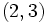# Sylow not implies CDIN

This article gives the statement and possibly, proof, of a non-implication relation between two subgroup properties. That is, it states that every subgroup satisfying the first subgroup property (i.e., Sylow subgroup) need not satisfy the second subgroup property (i.e., CDIN-subgroup)
View a complete list of subgroup property non-implications | View a complete list of subgroup property implications
EXPLORE EXAMPLES YOURSELF: View examples of subgroups satisfying property Sylow subgroup but not CDIN-subgroup|View examples of subgroups satisfying property Sylow subgroup and CDIN-subgroup

## Statement

We can have a group$G$ with a Sylow subgroup$P$ and elements$x,y \in P$ such that$x$ and$y$ are conjugate in$G$ but not in$N_G(P)$. In other words,$P$ is not a CDIN-subgroup.

## Related facts

• Sylow and TI implies CDIN: If we assume the additional condition that the Sylow subgroup intersects all its distinct conjugates trivially, then any two elements of it that are conjugate in the whole group are, in fact, conjugate in its normalizer.

## Proof

### Example of the symmetric group of degree four

Further information: symmetric group:S4, dihedral group:D8

Let$G$ be the symmetric group of degree four, say, on the set$\{ 1,2,3,4 \}$. Let$P$ be a$2$-Sylow subgroup of$G$, say:$P = \{ (), (1,2,3,4), (1,3)(2,4), (1,4,3,2), (1,3), (2,4), (1,2)(3,4), (1,3)(2,4) \}$.

Note that$P$ is a$2$-Sylow subgroup of$G$, and is a dihedral group of order eight. Consider the elements of$P$ given by:$x = (1,3)(2,4), \qquad y = (1,2)(3,4)$.

•$x$ and$y$ are conjugate in$G$: The element$(2,3)$, for instance, conjugates$x$ to$y$.
•$P = N_G(P)$: Since$P$ has index three in$G$, either$N_G(P) = P$ or$N_G(P) = G$. But the element$(1,2)$, for instance, does not normalize$P$. This forces$N_G(P) = P$.
•$x$ and$y$ are not conjugate in$P = N_G(P)$: In fact,$x$ is in the center of$P$, and$y$ is not in the center of$P$.